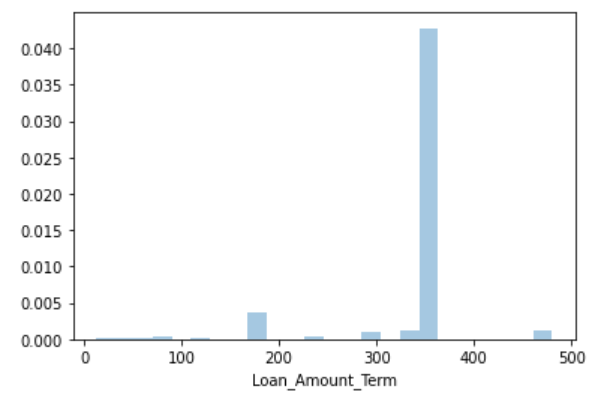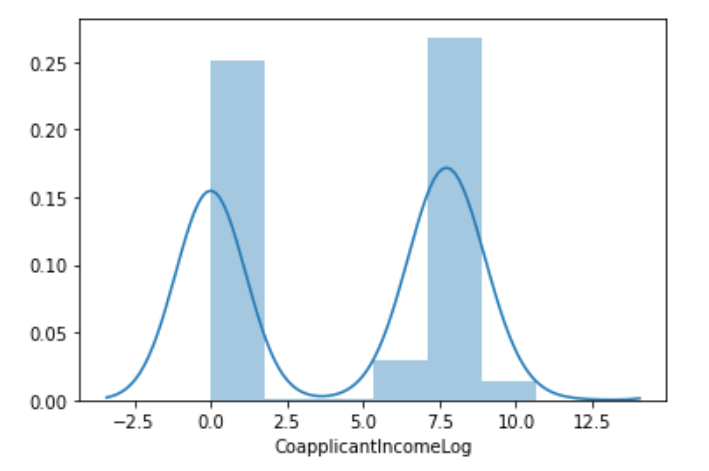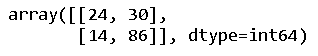top of page
• Hackers Realm

# Loan Prediction Analysis using Python | Classification | Machine Learning Project Tutorial

Updated: Jun 3

Unlock the power of loan prediction with Python! This tutorial explores classification techniques and machine learning algorithms to analyze and predict loan approvals. Learn to preprocess data, handle missing values, select meaningful features, and build models that can accurately predict loan outcomes. Enhance your skills in data preprocessing, feature engineering, machine learning, and contribute to informed decision-making in the lending industry. Join this comprehensive project tutorial to unravel the complexities of loan prediction and become proficient in using Python for classification tasks. #LoanPrediction #Python #Classification #MachineLearning #DataPreprocessing #FeatureEngineeringLoan Prediction Analysis

In this project tutorial, we are learning about Loan Prediction and its Analysis in Python. It is a classification problem. The objective of this problem is to predict whether the loan will approve or not.

You can watch the video-based tutorial with step by step explanation down below.

## Dataset Information

Dream Housing Finance company deals in all home loans. They have a presence across all urban, semi-urban and rural areas. Customer-first applies for a home loan after that company validates the customer's eligibility for a loan. The company wants to automate the loan eligibility process (real-time) based on customer detail provided while filling out the online application form.

These details are Gender, Marital Status, Education, Number of Dependents, Income, Loan Amount, Credit History and others. To automate this process, they have given a problem to identify the customer's segments, those are eligible for loan amount so that they can specifically target these customers.

This is a standard supervised classification task. A classification problem where we have to predict whether a loan would be approved or not. Below is the dataset attributes with a description.Loan Prediction Dataset

## Import Modules

First, we have to import all the basic modules we will be needing for this project.

```import pandas as pd
import numpy as np
import seaborn as sns
from matplotlib import pyplot as plt
import matplotlib
%matplotlib inline
import warnings
warnings.filterwarnings('ignore')```
• pandas - used to perform data manipulation and analysis

• numpy - used to perform a wide variety of mathematical operations on arrays

• matplotlib - used for data visualization and graphical plotting

• seaborn - built on top of matplotlib with similar functionalities

• %matplotlib - to enable the inline plotting.

• warnings - to manipulate warnings details filterwarnings('ignore') is to ignore the warnings thrown by the modules (gives clean results)

```df = pd.read_csv("Loan Prediction Dataset.csv")Loan Prediction Dataset
• We have to predict the output variable "Loan status".

• The Input attributes are in categorical as well as in numerical form.

• We have to analyze all the attributes.

## Statistics Data Information

`df.describe()`Statistical Information of Dataset
• The total count column displays some missing values, which we will deal with later.

• The credit history attributes are in the range of 0 to 1.

`df.info()`Data type Information of Dataset
• We can observe 13 attributes. Out of which 4 attributes are in float, 1 attribute is in integer and the other 8 are in objects.

• We can change the object into corresponding data to reduce the usage memory.

• However, we have 62 KB of memory usage, therefore we don't have to change any of the data types.

## Preprocessing the Loan Sanction Data

Let us check for NULL values in the dataset.

```# find the null values
df.isnull().sum()```Number of NULL Values
• We have found 6 columns having NULL values.

• Now, we have to replace the NULL values with some common values.

Let us fill in the missing values for numerical terms using mean.

```# fill the missing values for numerical terms - mean
df['LoanAmount'] = df['LoanAmount'].fillna(df['LoanAmount'].mean())
df['Loan_Amount_Term'] = df['Loan_Amount_Term'].fillna(df['Loan_Amount_Term'].mean())
df['Credit_History'] = df['Credit_History'].fillna(df['Credit_History']```
• All the missing values will be filled with the mean of the current column.

Let us now fill in the missing values for categorical terms using mode operation.

```# fill the missing values for categorical terms - mode
df['Gender'] = df["Gender"].fillna(df['Gender'].mode())
df['Married'] = df["Married"].fillna(df['Married'].mode())
df['Dependents'] = df["Dependents"].fillna(df['Dependents'].mode())
df['Self_Employed'] = df["Self_Employed"].fillna(df['Self_Employed'].mode())```
• All the missing values will be filled with the most frequently occurring values.

• Modes give the result in their terms of the data frame, so we only need the values. We will specify 0th index to display the values.

Now, let's check for the NULL values again.

`df.isnull().sum()`• All the NULL values are now replaced.

## Exploratory Data Analysis

Let us first explore the categorical column "Gender".

```# categorical attributes visualization
sns.countplot(df['Gender'])```Distribution of Gender
• The majority of the applicant is male and a handful is female.

• From these analyses, we will get an intuition that will be useful in building the model.

To display the column "Married".

`sns.countplot(df['Married'])`Distribution of Married
• The majority of the applicants are married.

To display the column "Dependents".

`sns.countplot(df['Dependents'])`Distribution of Dependents
• The majority of the applicants have zero dependents, around 100 applicants have one or two dependents and only a few have more than three dependents.

To display the column "Education".

`sns.countplot(df['Education'])`Distribution of Education

To display the column "Self Employed".

`sns.countplot(df['Self_Employed'])`Distribution of Self Employed
• Around 90 applicants are either freelancers or run a business.

To display the column "Property Area".

`sns.countplot(df['Property_Area'])`Distribution of Property Area
• We can assume that the applicants are equally distributed in urban, rural and semi-urban areas.

To display the column "Loan Status".

`sns.countplot(df['Loan_Status'])`Distribution of Loan Status
• Around 400 loans are accepted and 200 loans are rejected. Its shows the 2:1 ratio.

Let us first explore the Numerical column "Applicant Income".

```# numerical attributes visualization
sns.distplot(df["ApplicantIncome"])```Distribution of Applicant Income
• The data are skewed left in the graph, which is not a suitable distribution to train a Model.

• Hence, we will apply the Log Transformation later to normalize the attributes in the form of Bell Curve (Normal Distribution).

To display the column "Co-applicant Income".

`sns.distplot(df["CoapplicantIncome"])`Distribution of Co-applicant Income
• We have to normalize this graph as well.

To display the column "Loan Amount".

`sns.distplot(df["LoanAmount"])`Distribution of Loan Amount
• The loan amount graph is slightly right-skewed. We will consider this for Normalization.

To display the column "Loan Amount Term".

`sns.distplot(df['Loan_Amount_Term'])`Distribution of Loan Amount Term
• The majority of them are filled will main values, that is the highest values. We will apply log transformation of this as well.

To display the column "Credit History".

`sns.distplot(df['Credit_History'])`Distribution of Credit History
• Since the values of credit history are in the range of 0 to 1, we don't need to normalize this graph.

## Creation of new attributes

We can create a new attribute performing Log Transformation. We can also create a new attribute Total Income, that is the sum of Applicant Income and Co-applicant Income.

```# total income
df['Total_Income'] = df['ApplicantIncome'] + df['CoapplicantIncome']Creation of Total Income Attribute

## Log Transformation

Log transformation helps to make the highly skewed distribution to less skewed. Instead of changing the column, we will add the data into a new column by writing 'Log' after each column.

To display the column "Applicant Income Log".

```# apply log transformation to the attribute
df['ApplicantIncomeLog'] = np.log(df['ApplicantIncome']+1)
sns.distplot(df["ApplicantIncomeLog"])```Applicant Income after Log Transformation
• We can observe a Normal distribution in a form of a Bell Curve.

To display the column "Co-applicant Income Log".

```df['CoapplicantIncomeLog'] = np.log(df['CoapplicantIncome']+1)
sns.distplot(df["CoapplicantIncomeLog"])```Co-applicant Income after Log Transformation

To display the column "Loan Amount Log".

```df['LoanAmountLog'] = np.log(df['LoanAmount']+1)
sns.distplot(df["LoanAmountLog"])```Loan Amount after Log Transformation

To display the column "Loan Amount Term Log".

```df['Loan_Amount_Term_Log'] = np.log(df['Loan_Amount_Term']+1)
sns.distplot(df["Loan_Amount_Term_Log"])```Loan Amount Term after Log Transformation
• The Loan amount term is slightly better than before. Despite the fact that it is skewed right.

To display the column "Total Income Log".

```df['Total_Income_Log'] = np.log(df['Total_Income']+1)
sns.distplot(df["Total_Income_Log"])```Total Income after Log Transformation
• We can observe the normal distribution of the newly created column 'Total Income'.

After normalizing all the data in the dataset, let's check the correlation matrix.

## Correlation Matrix

For this project, the correlation matrix will discover the correlation for numerical attributes.

```corr = df.corr()
plt.figure(figsize=(15,10))
sns.heatmap(corr, annot = True, cmap="BuPu")```Correlation Matrix
• In this graph, the higher density is plotted with dark color and the lower density is plotted with light color.

• We need to remove the highly correlated attributes.

• It means the original attributes are correlated with log attributes.

• We will remove the previous attributes and keep the log attributes to train our model.

To check the values of the dataset.

`df.head()`Let us drop some unnecessary columns.

```# drop unnecessary columns
cols = ['ApplicantIncome', 'CoapplicantIncome', "LoanAmount", "Loan_Amount_Term", "Total_Income", 'Loan_ID', 'CoapplicantIncomeLog']
df = df.drop(columns=cols, axis=1)• Out of all previous columns, we will keep 'Credit History'.

## Label Encoding

We will use label encoding to convert the categorical column into the numerical column.

```from sklearn.preprocessing import LabelEncoder
cols = ['Gender', "Married", "Education", 'Self_Employed', "Property_Area", "Loan_Status", "Dependents"]
le = LabelEncoder()
for col in cols:
df[col] = le.fit_transform(df[col])```
• We access each column from the column list. And for the corresponding column, the 'le.fit_transform()' function will convert the values into numerical then store them into the corresponding column.

`df.head()`• All the values of the dataset are now in numerical format. It will help us to train our model easily.

• For Loan status 1 indicates 'Yes' and 0 indicates 'No'.

## Splitting the data for Training and Testing

Before training and testing, we have to specify the input and output attributes.

```# specify input and output attributes
X = df.drop(columns=['Loan_Status'], axis=1)
y = df['Loan_Status']```

Let us now split the data.

```from sklearn.model_selection import train_test_split
x_train, x_test, y_train, y_test = train_test_split(X, y, test_size=0.25, random_state=42)```
• We will add random_state with the attribute 42 to get same split upon re-running.

• If you don't specify random state, it will randomly split the data upon re-running giving inconsistent results.

## Model Training

```# classify function
from sklearn.model_selection import cross_val_score
def classify(model, x, y):
x_train, x_test, y_train, y_test = train_test_split(X, y, test_size=0.25, random_state=42)
model.fit(x_train, y_train)
print("Accuracy is", model.score(x_test, y_test)*100)
# cross validation - it is used for better validation of model
# eg: cv-5, train-4, test-1
score = cross_val_score(model, x, y, cv=5)
print("Cross validation is",np.mean(score)*100)```
• Here, cross-validation will split the data set into multiple parts.

• For example; cv=5 means, it will split the data into 5 parts.

• For each iteration, the training will use 4 parts and testing will use 1 part.

• You can change the cross-validation with the common term 3 or 5.

Logistic Regression:

```from sklearn.linear_model import LogisticRegression
model = LogisticRegression()
classify(model, X, y)```• Since cross-validation deals with multiple parts, we have to focus on cross-validation percentage, which is an overall accuracy of the model.

Decision Tree:

```from sklearn.tree import DecisionTreeClassifier
model = DecisionTreeClassifier()
classify(model, X, y)```• The decision tree does not show good results.

Random Forest:

```from sklearn.ensemble import RandomForestClassifier,ExtraTreesClassifier
model = RandomForestClassifier()
classify(model, X, y)```• Random forest shows better results than a Decision tree.

Extra Trees:

```model = ExtraTreesClassifier()
classify(model, X, y)```• For this project, Extra tree doesn't show better results than random forest.

Out of all the classifiers, Logistic Regression shows a better result in terms of cross-validation. Now let's try to change some hyperparameters to improve the accuracy.

## Hyperparameter tuning

We will change some hyperparameters for Random Forest Classifiers.

```model = RandomForestClassifier(n_estimators=100, min_samples_split=25, max_depth=7, max_features=1)
classify(model, X, y)```• Generally, we change the parameter with the use of algorithms like Grid Search and Random Search.

• You can also use any algorithm of your convenience.

## Confusion Matrix

A confusion matrix is a summary of prediction results on a classification problem. The number of correct and incorrect predictions are summarized with count values and broken down by each class. It gives us insight not only into the errors being made by a classifier but more importantly the types of errors that are being made.

We will use the Random Forest Model.

```model = RandomForestClassifier()
model.fit(x_train, y_train)```

After running the basic default parameters we will plot the confusion matrix.

```from sklearn.metrics import confusion_matrix
y_pred = model.predict(x_test)
cm = confusion_matrix(y_test, y_pred)
cm```• y_test contains the actual values from the dataset.

• y_predict contains the predicted values from the model.

To display the confusion matrix in a heat map.

`sns.heatmap(cm, annot=True)`Confusion Matrix

The left side of the heatmap indicates actual values, and the bottom side shows predicted values.

• For actual value '0' there are 24 correct predictions. For actual value '1' there are 86 correct predictions.

• The model has falsely predicted 30 counts for class 0. Therefore, we need to train better for class 0.

Similarly, we can compose other additional assumptions from the confusion matrix.

## Final Thoughts

• To summarize, the left diagonal shows the correctly predicted counts/numbers. And the right diagonal shows the inaccurately predicted counts/numbers.

• For multiple classes, the matrix will be the n*n matrix. Here, n is the number of output classes.

In this article, we have analyzed the dataset for loan prediction using machine learning. Apart from this, we have discussed the importance of a confusion matrix and also consider different classifiers to train the data.

Get the project notebook from here